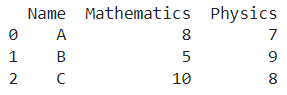# How to convert Pandas Dataframe To Dictionary?

A DataFrame is the primary data structure of the Pandas library in Python and is commonly used for storing and working with tabular data.

A common operation that could be performed on such data is to convert a Pandas dataframe to a dictionary in order to have the information in a more workable format.

To start working with Pandas, we first need to import it :

``import pandas as pd``

## Running Example

Let us understand this operation with the help of an example. Consider the following DataFrame containing 3 students with names A, B, and C and their corresponding marks (out of 10) for two subjects, Mathematics and Physics.Code snippet for generating the above DataFrame :

### Python 3 Code :

``````import pandas as pd

# Dictionary for our data
data = {'Name' : ['A', 'B', 'C'], 'Mathematics' : [8, 5, 10], 'Physics' : [7, 9, 8]}

# DataFrame for the dictionary
df = pd.DataFrame(data)

# Printing the DataFrame
print(df)``````

Here, data is a dictionary we created to initialize the DataFrame.

For this, we use the DataFrame() function of the Pandas library which takes the dictionary as an argument and returns the required DataFrame.

Now, let’s say we need to convert this dataframe into a dictionary to maybe have the information in a more tractable format.

The resulting output would look like this :

``{'Name': {0: 'A', 1: 'B', 2: 'C'}, 'Mathematics': {0: 8, 1: 5, 2: 10}, 'Physics': {0: 7, 1: 9, 2: 8}}``

Let us look at different ways of performing this operation on a given DataFrame :

## 1. Using the DataFrame.to_dict() function to convert dataframe to a dictionary in Python

In this method, we use the DataFrame.to_dict() function to convert the given dataframe into the desired form, like a dictionary.

The resultant dictionary is obtained as the returned object.

If we do not pass in any parameter, then by default, a dictionary is returned which contains column labels as keys and corresponding values as dictionaries which in turn contain index-data pairs as key-value pairs.

This is equivalent to passing the parameter as dict.

The changes are not made in place so reassignment is required.  Let us take a look at the corresponding code snippet and generated output for this method:

``````# Importing required libraries
import pandas as pd

# Dictionary for our data
data = {'Name' : ['A', 'B', 'C'], 'Mathematics' : [8, 5, 10], 'Physics' : [7, 9, 8]}

# DataFrame for the dictionary
df = pd.DataFrame(data=data)

# Performing the operation
df = df.to_dict()

# Printing the result
print(df)``````

Output :

{'Name': {0: 'A', 1: 'B', 2: 'C'}, 'Mathematics': {0: 8, 1: 5, 2: 10}, 'Physics': {0: 7, 1: 9, 2: 8}}

Instead of passing the parameter as dict, if we need to have the dictionary contain keys as column labels and values as lists containing row-wise data, we pass the parameter as a list.

The changes are not made in place so reassignment is required.

Let us take a look at the corresponding code snippet and generated output for this method :

``````# Importing required libraries
import pandas as pd

# Dictionary for our data
data = {'Name' : ['A', 'B', 'C'], 'Mathematics' : [8, 5, 10], 'Physics' : [7, 9, 8]}

# DataFrame for the dictionary
df = pd.DataFrame(data=data)

# Performing the operation
df = df.to_dict('list')

# Printing the result
print(df)``````

Output :

{'Name': ['A', 'B', 'C'], 'Mathematics': [8, 5, 10], 'Physics': [7, 9, 8]}

Instead of passing the parameter as dict, if we pass it as split, we have data in a different format.

The keys of the resulting dictionary are index, columns, and data.

The index contains the index labels, the column has the column labels whereas data contains row-wise data.

The changes are not made in place so reassignment is required.

Let us take a look at the corresponding code snippet and generated output for this method :

``````# Importing required libraries
import pandas as pd

# Dictionary for our data
data = {'Name' : ['A', 'B', 'C'], 'Mathematics' : [8, 5, 10], 'Physics' : [7, 9, 8]}

# DataFrame for the dictionary
df = pd.DataFrame(data=data)

# Performing the operation
df = df.to_dict('split')

# Printing the result
print(df)``````

Output :

{'index': [0, 1, 2], 'columns': ['Name', 'Mathematics', 'Physics'], 'data': [['A', 8, 7], ['B', 5, 9], ['C', 10, 8]]}

## 2. Using the dict() function to convert dataframe to a dictionary in Python

In this method, we use the dict() function to convert the required Pandas dataframe to a dictionary.

The dataframe is passed as a parameter to this function and the desired dictionary is the returned object.

Let us take a look at the corresponding code snippet and generated output for this method:

``````# Importing required libraries
import pandas as pd

# Dictionary for our data
data = {'Name' : ['A', 'B', 'C'], 'Mathematics' : [8, 5, 10], 'Physics' : [7, 9, 8]}

# DataFrame for the dictionary
df = pd.DataFrame(data=data)

# Performing the operation
df = dict(df)

# Printing the result
print(df)``````

Output :

{'Name': 0    A

1    B

2    C

Name: Name, dtype: object, 'Mathematics': 0     8

1     5

2    10

Name: Mathematics, dtype: int64, 'Physics': 0    7

1    9

2    8

Name: Physics, dtype: float64}

## Conclusion

In this topic, we have learned to convert an existing Pandas DataFrame to a dictionary, following a running example of test scores of students in different subjects, thus giving us an intuition of how this concept could be applied in real-world situations. Feel free to reach out to info.javaexercise@gmail.com in case of any suggestions.

Trending